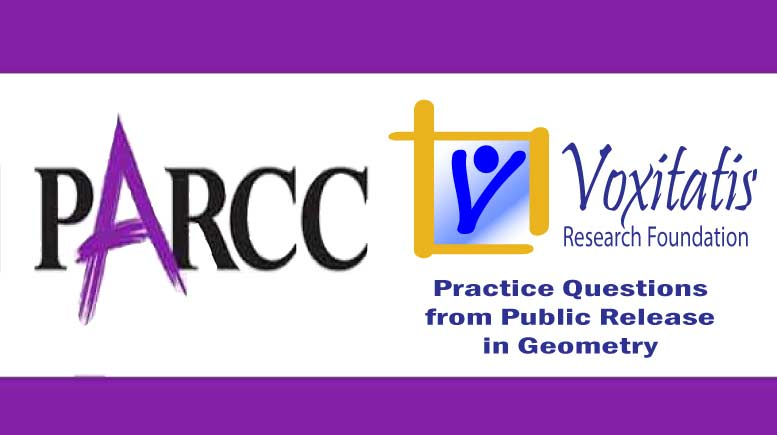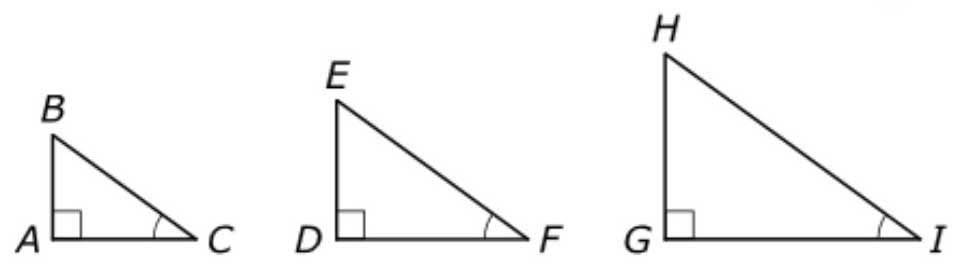Friday, June 18, 2021# Geometry PARCC question: similar triangles

-

#### The following fill-in-the-blanks question, explained here in hopes of helping geometry students in Maryland and Illinois prepare for the PARCC test near the end of this school year, appears on the released version of PARCC’s Spring 2015 test in geometry, here:In the three right triangles shown,$m\angle{C} = m\angle{F} = m\angle{I} = 36^{\circ}$

Complete the statements about the triangles by dragging the correct choices into the proper locations. Not all choices will be used.

Choices: AA similarity, adjacent leg, ASA, congruent, cosine, CPCTC, GH/GI, GI/GH, hypotenuse, similar, sine, tangent.

Because each triangle contains a right angle and a 36° angle, the triangles are ____ by ____, and AB/AC = DE/DF = ____. The proportion shows that the ratio of the length of the leg opposite the 36° angle to the length of the ____ will be the same for any right triangle with a 36° angle. The value of the ratio is defined to be the ____ of 36°.

Answer and references

Correct answers: similar … AA similarity … GH/GI … adjacent leg … tangent.

PARCC evidence statement(s) tested: G-SRT.6:

Understand that by similarity, side ratios in right triangles are properties of the angles in the triangle, leading to definitions of trigonometric ratios for acute angles.

Trigonometric ratios include sine, cosine, and tangent only.

The evidence statement above references Math Practice 7 in the Common Core:

[MP.7] Attend to precision. Mathematically proficient students look closely to discern a pattern or structure. Young students, for example, might notice that three and seven more is the same amount as seven and three more, or they may sort a collection of shapes according to how many sides the shapes have. Later, students will see 7 × 8 equals the well remembered 7 × 5 + 7 × 3, in preparation for learning about the distributive property. In the expression x2 + 9x + 14, older students can see the 14 as 2 × 7 and the 9 as 2 + 7. They recognize the significance of an existing line in a geometric figure and can use the strategy of drawing an auxiliary line for solving problems. They also can step back for an overview and shift perspective. They can see complicated things, such as some algebraic expressions, as single objects or as being composed of several objects. For example, they can see 5 – 3(xy)2 as 5 minus a positive number times a square and use that to realize that its value cannot be more than 5 for any real numbers x and y.

The question tests students’ understanding of the high school Common Core geometry standard HSG.SRT.C.6, found under High School: Geometry: Similarity, Right Triangles, & Trigonometry (define trigonometric ratios and solve problems involving right triangles), which states that they should “understand that by similarity, side ratios in right triangles are properties of the angles in the triangle, leading to definitions of trigonometric ratios for acute angles.” It also requires knowledge of other standards in this section of the Common Core, including HSG.SRT.A.3, which says they should be able to “use the properties of similarity transformations to establish the AA criterion for two triangles to be similar.”

## Resources for further study

Integrated Publishing begins a small proof that two right triangles that share the measure of one of the acute angles are similar to each other:

1. The right angle in the first triangle is equal to the right angle in the second, since all right angles are equal.
2. The sum of the angles of any triangle is 180°. Therefore, the sum of the two acute angles in a right triangle is 90°.

At the point, we note that, having shown that two of the angles have the same measure, AA (Angle-Angle) similarity applies. If two angles are equal in two different triangles, then the triangles are similar. The triangles would be congruent if the side between the two congruent angles were also congruent, by ASA (Angle-Side-Angle) congruency, but that’s not the case here.

For the second part, there are numerous sources on the Web and in textbooks to explain that the tangent of an angle in a right triangle is equal to the ratio of the side opposite the angle to the side adjacent to the angle, neither side being the hypotenuse of the right triangle.

Chapter 8, Section 8.3, of the book Geometry for Enjoyment and Challenge by Richard Rhoad et al, all teachers from Illinois, says the AA similarity theorem follows directly from the No-Choice theorem: If two angles of one triangle are congruent to two angles of a second triangle, then the third angles are congruent.

(Richard Rhoad, George Milauskas, and Robert Whipple. Geometry for Enjoyment and Challenge, new edition. Evanston, Ill.: McDougal Littell, a division of Houghton Mifflin Company, 1991. The book is used in several geometry classes taught in Illinois high schools.)

## Analysis of this question and online accessibility

The question measures knowledge of the Common Core standards it purports to measure and tests students’ understanding of the definition of a circle and its radius. It is considered to have a low cognitive demand.

The question can be tested online and should yield results that are as valid and reliable as those obtained on paper.

No special accommodation challenges can be identified with this question, so the question is considered fair.

## ChallengeGiven that line segment LP is perpendicular to line segment EA, N is the midpoint of line segment LP, and P and R trisect line segment EA, prove that$\Delta\textrm{PEN} \sim \Delta\textrm{PAL}$

## Purpose of this series of posts

Voxitatis is developing blog posts that address every algebra 1 question released to the public by the Partnership for Assessment of Readiness for College and Careers, or PARCC, in order to help students prepare to take the test this spring.

Our total release will run from February 27 through March 15, with one or two questions discussed per day. Then we’ll move to geometry at the end of March, algebra 2 during the first half of April, and eighth grade during the last half of April.Paul Katulahttps://news.schoolsdo.org
Paul Katula is the executive editor of the Voxitatis Research Foundation, which publishes this blog. For more information, see the About page.

### We congratulate the ‘Hybrid’ Class of 2021

0
We congratulate the 3 million or so seniors who graduate this spring amid a global pandemic and hope for a return to normal.